# Automata, equivalence of

(diff) ← Older revision | Latest revision (diff) | Newer revision → (diff)

An equivalence relation on the set of automata, which arises in the context of studying some individual internal properties of automata. Such a property is usually the behaviour of automata (cf. Automaton, behaviour of an), since two automata are considered equivalent if their behaviour is identical. In this context the behaviour of an automaton is usually understood to be the totality of functions realized by it (cf. Automaton, finite). For finite automata such an equivalence relation is solvable, and for this reason there exists a minimization algorithm for automata, i.e. an algorithm used to construct for a given automaton an equivalent automaton with the smallest possible number of states (a minimal automaton).

Equivalence of automata is conveniently defined in terms of the concept of equivalence of states: Two statesand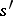of the automataand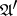(they may be identical) are said to be equivalent if the initialized automata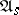and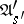realize the same function. Such an equivalence of the automataandwill then mean that it is possible to find, for an arbitrary state of, a state ofequivalent to it, and vice versa. An automaton is minimal if and only if no two states of it are equivalent. The minimal automaton is unambiguously defined for any automaton, up to an isomorphism. The algorithm of solution of this equivalence relation for finite automata is based on Moore's theorem, according to which statesandofare equivalent precisely if the functions realized by the initialized automataandare identical on words of length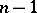, whereis the number of states of. If the statesandbelong, respectively, to two automataand, the estimate is equal to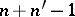, where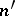is the number of states of. This theorem also forms the principle of a well-known minimization algorithm of finite automata, which involves the construction of the so-called reduced automaton, the states of which are classes of equivalent states, while its transition and output functions are naturally induced by the corresponding functions of the original automaton. A reduced automaton is minimal, since any two of its states are non-equivalent.

There exist asymptotic estimates of the number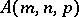of minimal automata withstates,input andoutput letters; if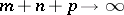for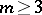, the estimate is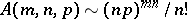, whileAnother problem in the study of equivalence of automata is the problem of equivalent transformations of automata. This problem was studied for two of the possible ways of defining finite automata — diagrams and logical networks. Generally speaking, the problem consists in finding a system of transformation rules which satisfy certain conditions and which enable one to transform any given automaton into an automaton equivalent to it: a so-called complete system of rules. In both cases the transformation rule is a pair of schemes (fragments of diagrams or logical networks) which realize identical choices of mappings. The rule is applied by substituting one fragment for another. For finite automata a complete system of rules cannot exist, but they do exist for logical networks with a limited number of delays.

The fundamental concepts, problems and methods which arise in the study of the equivalence of finite automata are usually extended to other types of automata, with due regard for their particular features. For references see Automaton.

The reader is referred to the articles Formal languages and automata and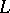-systems. Most of the topics in this article are nowadays not considered to be in the mainstream anymore.